# 2. (25 points) Compute the orbital inclination of an Earth satellite in a 500 km circular orbit w...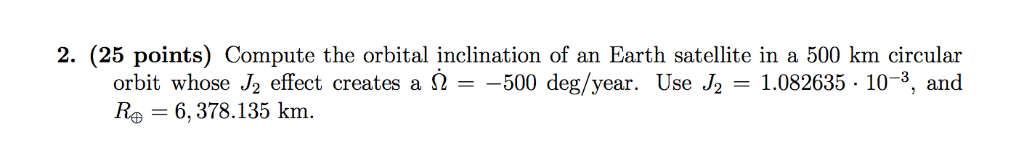2. (25 points) Compute the orbital inclination of an Earth satellite in a 500 km circular orbit whose J2 effect creates a 2-500 deg/year. Use J2 1.082635 - 10-3, and Re = 6,378.135 km.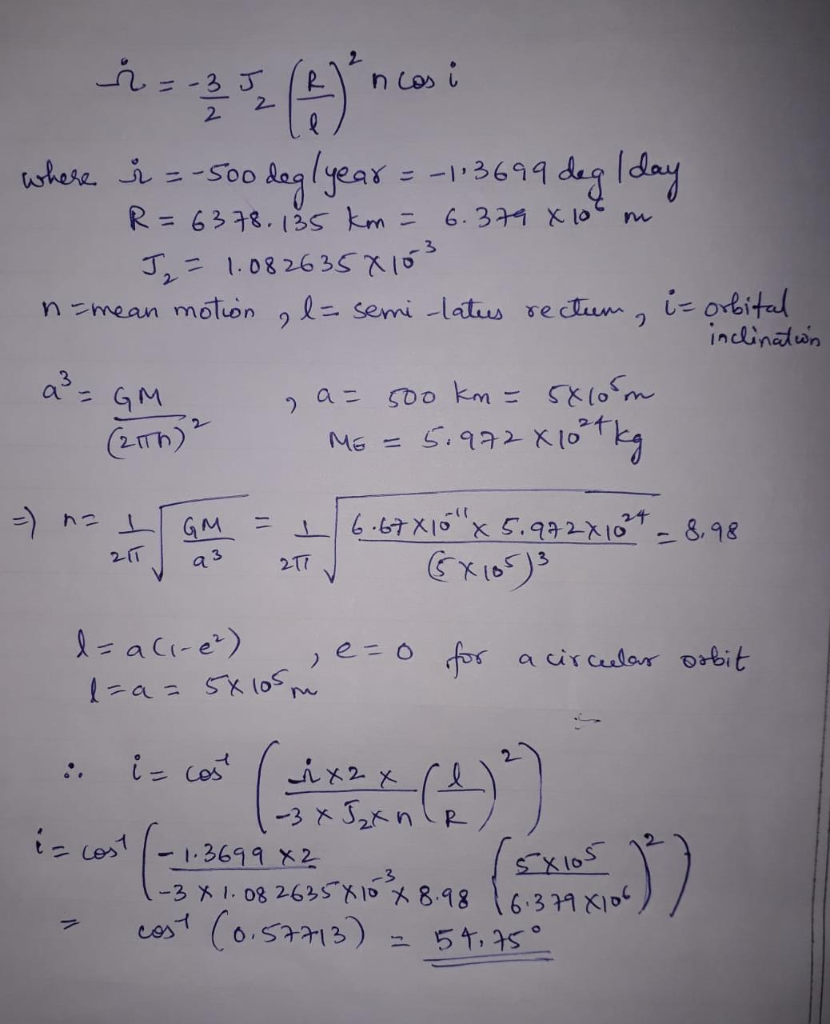##### Add Answer of: 2. (25 points) Compute the orbital inclination of an Earth satellite in a 500 km circular orbit w...
Similar Homework Help Questions
• ### An earth satellite moves in a circular orbit with an orbital speed of 5800 m/s

An earth satellite moves in a circular orbit with an orbital speed of 5800 m/s. Find the time (expressed in seconds) of one revolution of the satellite. Find the radial acceleration of the satellite in its orbit.I think we need to know the radius of the earth and the satellite's average distance from the earth in order to answer the question. All we have right now is the angular velocity. If you have that information, then use d=v*t where d...

• ### Problem 3) The space shuttle is in a circular orbit of 180 km altitude and inclination 30o. What ...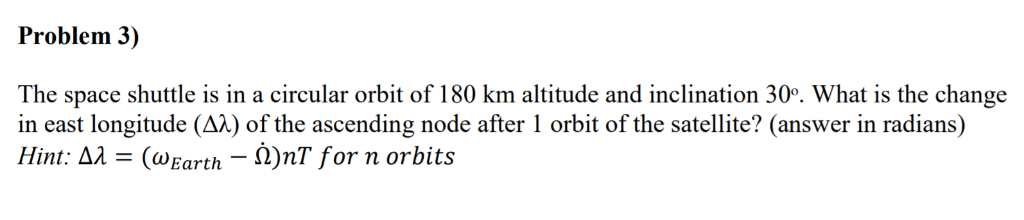Problem 3) The space shuttle is in a circular orbit of 180 km altitude and inclination 30o. What is the change in east longitude (AX) of the ascending node after 1 orbit of the satellite? (answer in radians) Hint: ёл-(w Earth-A)nT for n orbits Problem 3) The space shuttle is in a circular orbit of 180 km altitude and inclination 30o. What is the change in east longitude (AX) of the ascending node after 1 orbit of the satellite? (answer...

• ### Derive the "Clarke radius", the altitude above the surface of the Earth where a satellite in a circular orbit h...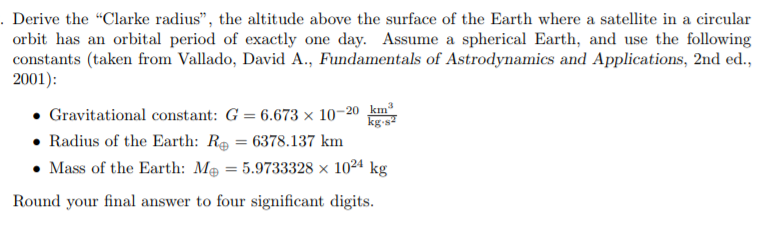Derive the "Clarke radius", the altitude above the surface of the Earth where a satellite in a circular orbit has an orbital period of exactly one day. Assume a spherical Earth, and use the following constants (taken from Vallado, David A., Fundamentals of Astrodynamics and Applications, 2nd ed. 2001) Gravitational constant: G 6.673 x 10-20 km Radius of the Earth: Re = 6378.137 km 1024 kg Mass of the Earth: Me = 5.9733328 x Round your final answer to four...

• ### For a satellite to be in a circular orbit 850 above the surface of the earth, what orbital speed...

Part AFor a satellite to be in a circular orbit 850 above the surface of the earth, what orbital speed must it be given?=___________m/s^2Part BWhat is the period of the orbit (in hours)?=___________hours

• ### A satellite of mass 210 kg is placed into Earth orbit at a height of 500...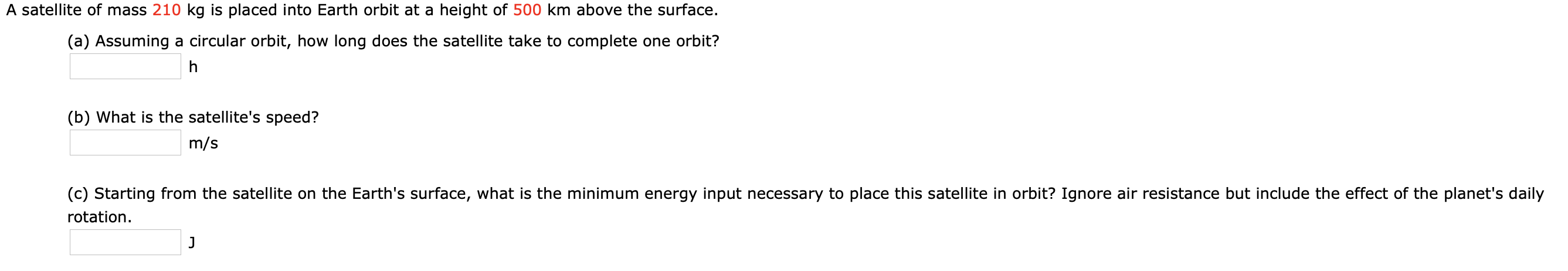A satellite of mass 210 kg is placed into Earth orbit at a height of 500 km above the surface. (a) Assuming a circular orbit, how long does the satellite take to complete one orbit? (b) What is the satellite's speed? m/s (C) Starting from the satellite on the Earth's surface, what is the minimum energy input necessary to place this satellite in orbit? Ignore air resistance but include the effect of the planet's daily rotation.

• ### An earth satellite moves in a circular orbit at a speed of 6234.87 m/s. What is its orbital...

An earth satellite moves in a circular orbit at a speed of 6234.87 m/s. What is its orbital period?

• ### The space shuttle releases a satellite into a circular orbit 550 km above the Earth

The space shuttle releases a satellite into a circular orbit 550 km above the Earth. How fast must the shuttle be moving (relative to Earth) when the release occurs?

• ### A 544-kg satellite is in a circular orbit about Earth at a height above Earth equal...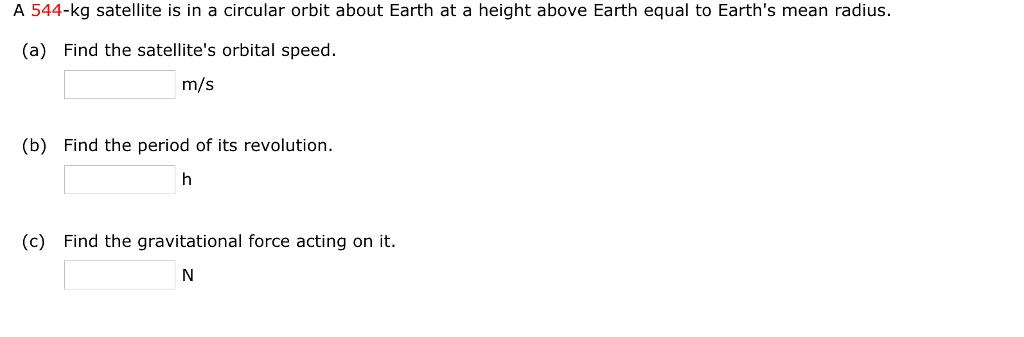A 544-kg satellite is in a circular orbit about Earth at a height above Earth equal to Earth's mean radius. (a) Find the satellite's orbital speed. m/s (b) Find the period of its revolution. (c) Find the gravitational force acting on it A satellite of Mars, called Phobos, has an orbital radius of 9.4 x 106 m and a period of 2.8 104 s. Assuming the orbit is circular, determine the mass of Mars. x 10 s. Assuming kg

• ### A 500 kg satellite is in a circular orbit at an altitude of 550 km above...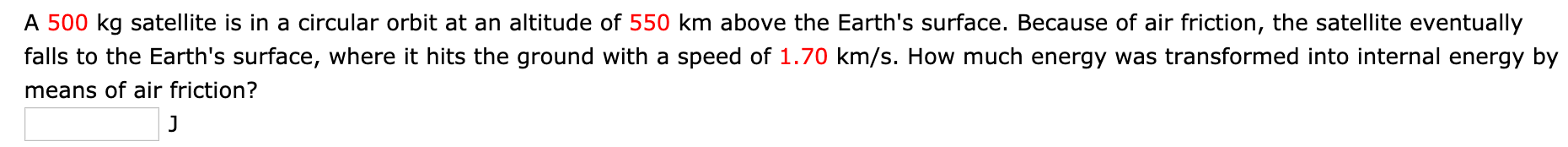A 500 kg satellite is in a circular orbit at an altitude of 550 km above the Earth's surface. Because of air friction, the satellite eventually falls to the Earth's surface, where it hits the ground with a speed of 1.70 km/s. How much energy was transformed into internal energy by means of air friction?

• ### A 500 kg satellite is in a circular orbit at an altitude of 450 km above...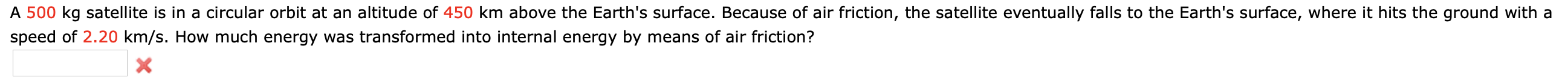A 500 kg satellite is in a circular orbit at an altitude of 450 km above the Earth's surface. Because of air friction, the satellite eventually falls to the Earth's surface, where it hits the ground with a speed of 2.20 km/s. How much energy was transformed into internal energy by means of air friction? X

Need Online Homework Help?